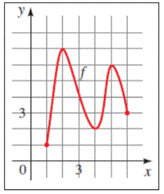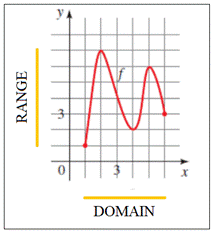# To fill in the gaps : with reference to the provided graph### Precalculus: Mathematics for Calcu...

6th Edition
Stewart + 5 others
Publisher: Cengage Learning
ISBN: 9780840068071### Precalculus: Mathematics for Calcu...

6th Edition
Stewart + 5 others
Publisher: Cengage Learning
ISBN: 9780840068071

#### Solutions

Chapter 2.3, Problem 2E
To determine

## To fill in the gaps: with reference to the provided graph

Expert Solution

The domain of the function f is all the x -values of the points on the graph, and the range is all the corresponding y -values on the graph. From the graph of f, we see that the domain of f is the interval [1, 6] and the range of f is the interval [1, 7] .

### Explanation of Solution

Given information: The domain of the function f is all the x -values of the points on the graph, and the range is all the corresponding y -values. From the graph of f, we see that the domain of f is the interval _____ and the range of f is the interval _____.From the provided information, consider,The domain of the graph of f is the interval from [1, 6] on the x -axis and the range of the graph is the interval from [1, 7] on the y- axis.

Hence, the domain of the function f is all the x -values of the points on the graph, and the range is all the corresponding y -values. From the graph of f, we see that the domain of f is the interval [1, 6] and the range of f is the interval [1, 7] .

### Have a homework question?

Subscribe to bartleby learn! Ask subject matter experts 30 homework questions each month. Plus, you’ll have access to millions of step-by-step textbook answers!